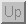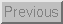Next: Problem: Noise Up: Data Acquisition Previous: Solution

## Analog to Digital Conversion

The purpose of the analog to digital is to quantize the input signal from the sample and hold circuit to 2B discrete levels - where B is the number of bits of the analog to digital converter (ADC). The input voltage can range from 0 to Vref (or -Vref to +Vref for a bipolar ADC). What this means is that the voltage reference of the ADC is used to set the range of conversion of the ADC. For a monopolar ADC, a 0V input will cause the converter to output all zeros. If the input to the ADC is equal to or larger thanthen the converter will output all ones. For inputs between these two voltage levels, the ADC will output binary numbers corresponding to the signal level. For a bipolar ADC, the minimum input isnot 0V.

Tim Stilson
Thu Oct 17 11:25:23 PDT 1996